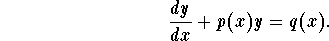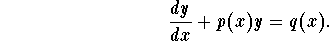# Linear differential equation problem

## Homework Statement

$$\ \frac{dy}{dx} + 2y = xe^x$$

## The Attempt at a Solution

I'v only ever solved differential equations where values can be seperated easily, i understand i may have to use something called the integrating factor but this does not seem to fit the formula layout ofThe equation you presented:

$$\frac{dy}{dx}+p(x)\cdot y=q(x)$$

is a linear differential equation of the first order. This is the next type you learn after the ones you can separate immediately. It has a general solution. What is your knowledge on the ways for solving these? You should have some idea about this, can you show an attempt of solving it?

Hurkyl
Staff Emeritus
Gold Member
$$\ \frac{dy}{dx} + 2y = xe^x$$
... does not seem to fit the formula layout ofWhy not? What doesn't match?

HallsofIvy